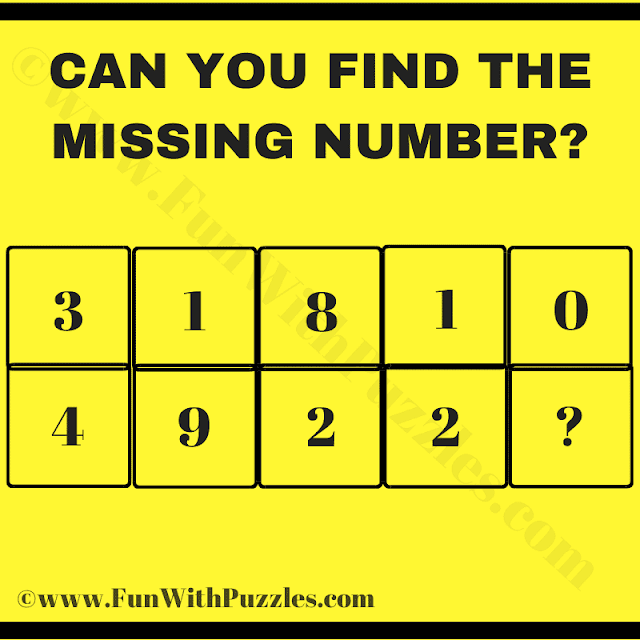This is a very interesting maths logic puzzle question that will twist your brain. This question is for Mathematical teachers, who can ask this puzzle question to their students. This puzzle will test your mathematical skills and logical reasoning ability. In this puzzle, you are shown some numbers in two different rows. There is one mathematical and logical formula that relates the given numbers. Can you crack the hidden logical code and find the value of the missing number which will replace the question mark. If you are able to solve this puzzle by looking at the answer, please post your answer in the comments along with the logical reasoning used to solve this puzzle question.Can you Find the Missing Number?

The answer to this "Tricky Maths Logic Puzzle Question", can be viewed by clicking on the answer button.